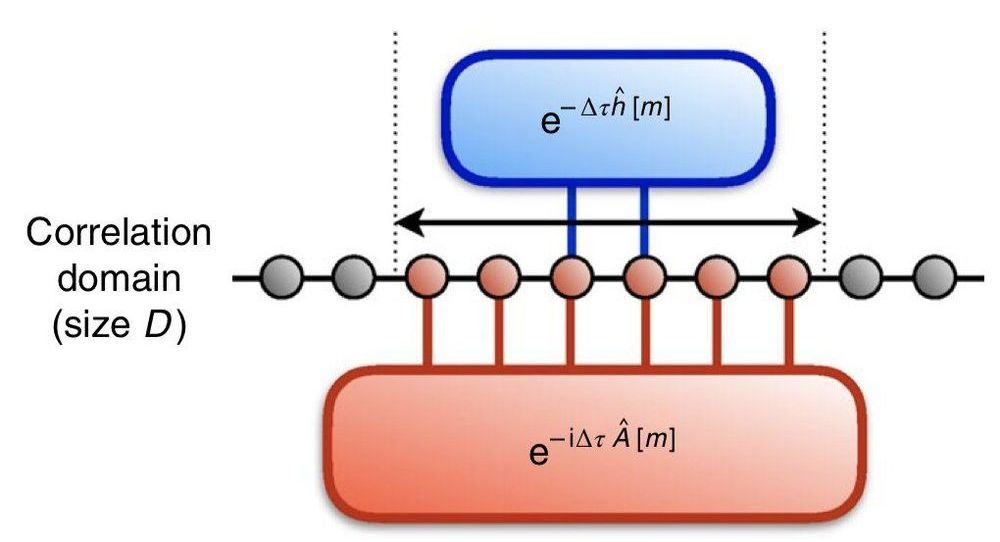Menu

## BlogDetermining the quantum mechanical behavior of many interacting particles is essential to solving important problems in a variety of scientific fields, including physics, chemistry and mathematics. For instance, in order to describe the electronic structure of materials and molecules, researchers first need to find the ground, excited and thermal states of the Born-Oppenheimer Hamiltonian approximation. In quantum chemistry, the Born-Oppenheimer approximation is the assumption that electronic and nuclear motions in molecules can be separated.

A variety of other scientific problems also require the accurate computation of Hamiltonian ground, excited and thermal states on a quantum computer. An important example are combinatorial optimization problems, which can be reduced to finding the ground state of suitable spin systems.

So far, techniques for computing Hamiltonian eigenstates on quantum computers have been primarily based on phase estimation or variational algorithms, which are designed to approximate the lowest energy eigenstate (i.e., ground state) and a number of excited states. Unfortunately, these techniques can have significant disadvantages, which make them impracticable for solving many scientific problems.